Author: Oscar Cronquist Article last updated on October 31, 2018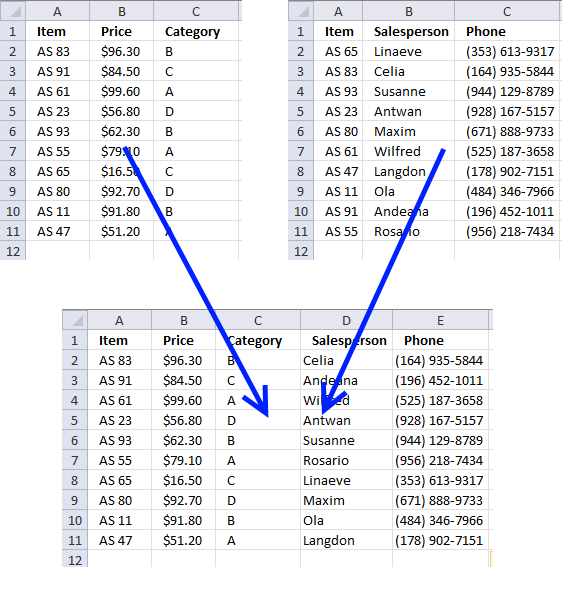I have written a post about merging two single columns or ranges before: Merge two columns with possible blank cells. It demonstrates how to merge two different cell ranges dynamically and that is it. The following examples merge data tables with a criterion or criteria.

Table of Contents

### Example 1 - Vlookup

Here are two lists on two different sheets. They only share the same items.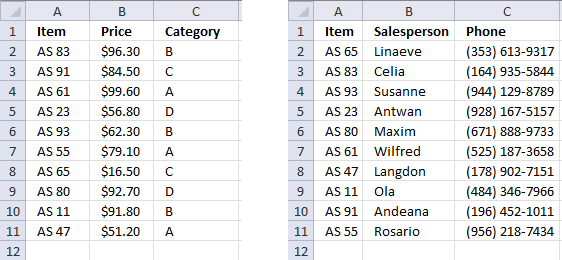Let us use the Item in the first list and search for it in the second list. The VLOOKUP function allows us to retrieve corresponding values. Here is the sheet1 list merged with sheet2 list.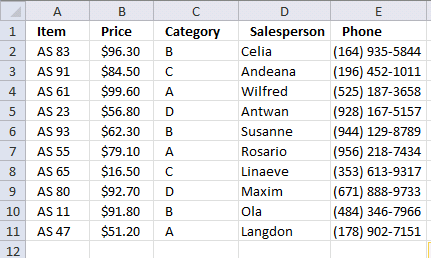Formula in cell D2:

=VLOOKUP(\$A2,'Ex 1 - Sheet2'!\$A\$2:\$C\$11,COLUMN(B1),FALSE)

Copy cell D2 and paste to E2. Then copy D2:E2 and paste to D3:E11.

Explaining formula

Step 1 - VLOOKUP function

VLOOKUP(lookup_valuetable_arraycol_index_num ,range_lookup)
The Vlookup function looks for a value in the leftmost column of a table and then returns a value in the same row from a column you specify.

Step 2 - lookup_value argument

The formula is entered in cell D2. It uses the lookup value in cell \$A2. See the \$ sign? It makes column A absolute, meaning it does not change when we copy the formula to column E.

Step 3 - table_array argument

The Vlookup function looks for the value in cell \$A2 in the leftmost column of cell range 'Ex 1 - Sheet2'!\$A\$2:\$C\$11. It also returns a value from this cell range if there is a match and depending on the chosen column number in argument col_index_num. See next step.

Step 4 - col_index_num argument

col_index_num is a column number determining which column in cell 'Ex 1 - Sheet2'!\$A\$2:\$C\$11 we want to get values from. In my example I use COLUMN(B1), it returns 2 for formulas in column D and 3 for formulas in column E.

Step 5 - range_lookup argument

TRUE - Approximate match
FALSE - Exact match

### Example 2 - INDEX and MATCH

Here are two lists on two different sheets. They share the same items. The second list has "Items" in column B (blue circle). You can't use the VLOOKUP function now unless you move the Items column to the leftmost column in the cell range. But wait, you don´t have to do that.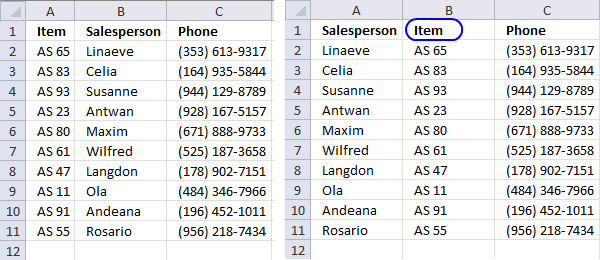This picture shows the merged list using INDEX and MATCH function.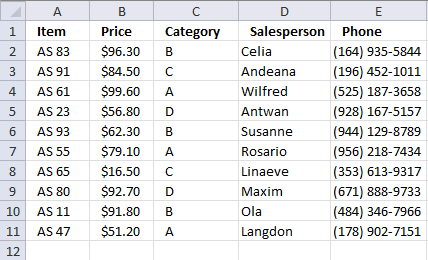Formula in cell D2:

=INDEX('Ex 2 - Sheet2'!\$A\$2:\$C\$11, MATCH('Ex 2 - Sheet1'!A2,'Ex 2 - Sheet2'!\$B\$2:\$B\$11, 0), 1)

Copy cell D2 to cell range D3:D11.

Formula in cell E2:

=INDEX('Ex 2 - Sheet2'!\$A\$2:\$C\$11, MATCH('Ex 2 - Sheet1'!A2,'Ex 2 - Sheet2'!\$B\$2:\$B\$11, 0), 3)

Copy cell E2 to cell range E3:E11.

Explaining fomula in cell D2

Step 1 - Find the relative position with the value in cell A2 in cell range 'Ex 2 - Sheet2'!\$B\$2:\$B\$11

MATCH('Ex 2 - Sheet1'!A2,'Ex 2 - Sheet2'!\$B\$2:\$B\$11,0)

becomes

MATCH("As 83",{"AS 65";"AS 83";"AS 93";"AS 23";"AS 80";"AS 61";"AS 47";"AS 11";"AS 91";"AS 55"},0)

and returns 2.

Step 2 - Return a value of the cell at the intersection of a particular row and column

=INDEX('Ex 2 - Sheet2'!\$A\$2:\$C\$11,MATCH('Ex 2 - Sheet1'!A2,'Ex 2 - Sheet2'!\$B\$2:\$B\$11,0),1)

becomes

=INDEX('Ex 2 - Sheet2'!\$A\$2:\$C\$11,2,1)

becomes

=INDEX({"Linaeve","AS 65","(353) 613-9317"; "Celia","AS 83","(164) 935-5844"; "Susanne","AS 93","(944) 129-8789"; "Antwan","AS 23","(928) 167-5157"; "Maxim","AS 80","(671) 888-9733"; "Wilfred","AS 61","(525) 187-3658"; "Langdon","AS 47","(178) 902-7151"; "Ola","AS 11","(484) 346-7966"; "Andeana","AS 91","(196) 452-1011"; "Rosario","AS 55","(956) 218-7434"},2,1)

and returns value "Celia" from row 2 and column 1 in cell range 'Ex 2 - Sheet2'!\$A\$2:\$C\$11.

### Example 3 - Wild card search

Here we want to find the color for each category. The second list contains multiple categories and a corresponding color.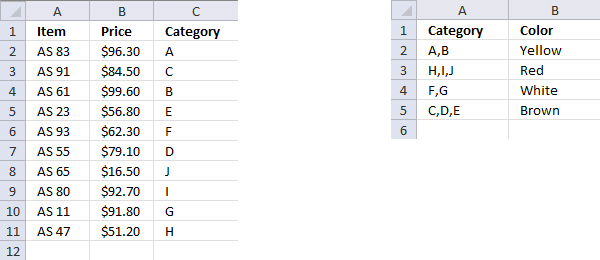This picture shows the merged list.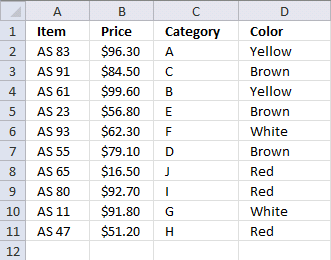Formula in cell D2:

=INDEX('Ex 3 - Sheet2'!\$B\$2:\$B\$5, MATCH("*"&C2&"*",'Ex 3 - Sheet2'!\$A\$2:\$A\$5, 0))

Copy cell D2 and paste it to cell range D3:D11.

To be honest, you can use the VLOOKUP function in this example also, as long as you search in the leftmost column:

=VLOOKUP("*"&C2&"*", 'Ex 3 - Sheet2'!\$A\$2:\$B\$5, 2, FALSE)

You can use other characters, as well: Wildcard characters

Explaining the array formula in cell D2

Step 1 - Search for a text string in an array of cell values and return relative position of first match in array

MATCH("*"&C2&"*",'Ex 3 - Sheet2'!\$A\$2:\$A\$5,0)

becomes

MATCH("*A*", {"A,B";"H,I,J";"F,G";"C,D,E"},0) and returns 1

Step 2 - Return a value of the cell at the intersection of a particular row and column

=INDEX('Ex 3 - Sheet2'!\$B\$2:\$B\$5,MATCH("*"&C2&"*",'Ex 3 - Sheet2'!\$A\$2:\$A\$5,0))

becomes

=INDEX({"Yellow"; "Red"; "White"; "Brown"}, 1)

and returns "Yellow" in cell D2.

### Example 4 - Match multiple columns

This example demonstrates how to match multiple values from two different columns. Both values must match.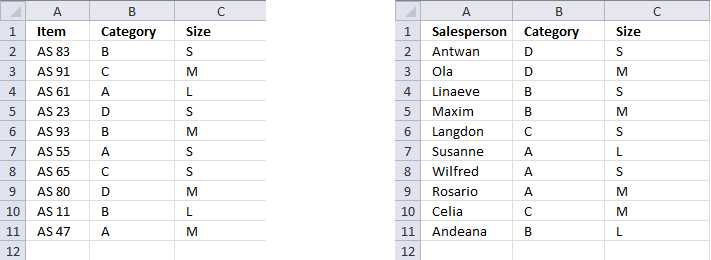Here is the merged list. The COUNTIFS function allows you to match multiple columns.Array formula in cell D2:

=INDEX('Ex 4 - Sheet2'!\$A\$2:\$A\$11, MATCH(1, COUNTIFS(B2, 'Ex 4 - Sheet2'!\$B\$2:\$B\$11, C2, 'Ex 4 - Sheet2'!\$C\$2:\$C\$11), 0))

This is an array formula. Type the formula in cell D2. Press and hold CTRL + SHFT simultaneously, then press ENTER once. Release all keys. If you did it right, there are now curly brackets before and after the formula.

Copy cell D2 and paste it to D3:D11.

Explaining array formula in cell D2

Step 1 - Check if value in cell B2 matches 'Ex 4 - Sheet2'!\$B\$2:\$B\$11 AND if value in cell C2 matches 'Ex 4 - Sheet2'!\$C\$2:\$C\$11

COUNTIFS(B2,'Ex 4 - Sheet2'!\$B\$2:\$B\$11,C2,'Ex 4 - Sheet2'!\$C\$2:\$C\$11)

becomes

COUNTIFS("B",{"D"; "D"; "B"; "B"; "C"; "A"; "A"; "A"; "C"; "B"},"S",{"S"; "M"; "S"; "M"; "S"; "L"; "S"; "M"; "M"; "L"})

and returns {0; 0; 1; 0; 0; 0; 0; 0; 0; 0}

Step 2 - Find the relative position of 1

MATCH(1,COUNTIFS(B2,'Ex 4 - Sheet2'!\$B\$2:\$B\$11,C2,'Ex 4 - Sheet2'!\$C\$2:\$C\$11),0)

becomes

MATCH(1,{0; 0; 1; 0; 0; 0; 0; 0; 0; 0},0)

and returns 3.

Step 3 - Return a value of the cell at the intersection of a particular row and column

INDEX('Ex 4 - Sheet2'!\$A\$2:\$A\$11, MATCH(1, COUNTIFS(B2, 'Ex 4 - Sheet2'!\$B\$2:\$B\$11, C2, 'Ex 4 - Sheet2'!\$C\$2:\$C\$11), 0))

becomes

INDEX('Ex 4 - Sheet2'!\$A\$2:\$A\$11, 3)

and returns "Linaeve"

### Download excel *.xlsx file

Merge lists with criteria.xlsx